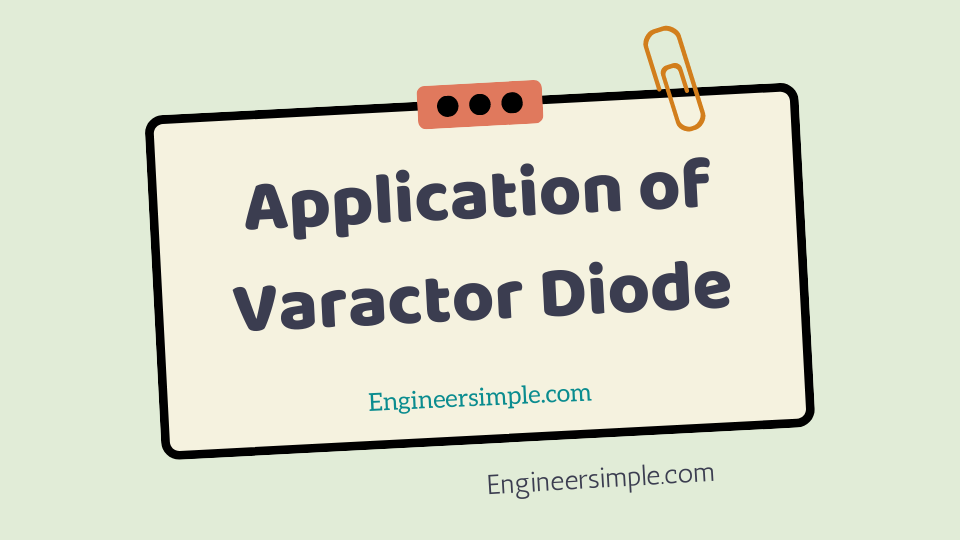Application of Varactor Diode - Engineer Simple

#A junction diode which acts as a variable capacitor under changing reverse bias is known as avaractor diode.When a pn junction is formed, depletion layer is created in the junction area. Since there are nocharge carriers within the depletion zone, the zone acts as an insulator. We have discussed that we can increase or decrease the junc-tion capacitance of varactor diode simply by changing thereverse bias on the diode. This makes a varactor diode idealfor use in circuits that require voltage-controlled tuning. Fig.1 shows the use of varactor diode in a tuned circuit.

#### Note that- the capacitance of the varactor is in parallel with theinductor. The varactor and the inductor form a parallel LCcircuit.

For normal operation, a varactor diode is always operate under reverse bias.In fact, this condition is metin the circuit show in Fig. 1.

The resistance RW in thecircuit is the winding resistance of the inductor. This winding resistance is in series with the potenti-ometer R1. Thus R1 and RW form a voltage divider that is use to determine the amount of reverse biasacross the varactor diode D1 and therefore its capacitance.application of varactor diode- By adjusting the setting of R1, we can varythe diode capacitance. This, in turn, varies the resonant frequency of the LC circuit. The resonant frequency fr of the LC circuit is give by;

If the amount of varactor reverse bias is decreased, the value of C of the varactor increases.Theincrease in C will cause the resonant frequency of the circuit to decrease.Thus, a decrease in reversebias causes a decrease in resonant frequency and vice-versa.Example 7.5.The LC tank circuit shown in Fig. 1 has a 1 mH inductor. The varactor hascapacitance of 100 pF when reverse bias is 5V d.c. Determine the resonant frequency of the circuitfor this reverse bias.Solution.

Resonant frequency,

Here, L = 1mH = 1 × 10−3 H; C = 100 pF = 100 × 10−12 F